CHIRAG GOYAL — Published On May 21, 2021 and Last Modified On August 5th, 2022

This article was published as a part of the Data Science Blogathon

## Introduction

In most of the real-life problem statements of Machine learning, it is very common that we have many relevant features available to build our model but only a small portion of them are observable. Since we do not have the values for the not observed (latent) variables, the Expectation-Maximization algorithm tries to use the existing data to determine the optimum values for these variables and then finds the model parameters.

• 👉 What is the Expectation-Maximization (EM) Algorithm?
• 👉 Detailed Explanation of EM Algorithm
• 👉 Flow chart
• 👉 Applications of EM Algorithm
• 👉 Use case of EM Algorithm
• Introduction to Gaussian Distributions
• Gaussian Mixture Models (GMMs)
• 👉  Implementation of Gaussian Mixture Models in Python

## What is Expectation-Maximization (EM) algorithm?

👉 It is a latent variable model.

Let’s first understand what is meant by the latent variable model?

A latent variable model consists of observable variables along with unobservable variables. Observed variables are those variables in the dataset that can be measured whereas unobserved (latent/hidden) variables are inferred from the observed variables.

• 👉 It can be used to find the local maximum likelihood (MLE) parameters or maximum a posteriori (MAP) parameters for latent variables
in a statistical or mathematical model.
• 👉 It is used to predict these missing values in the dataset, provided we know the general form of probability distribution associated with these      latent variables.
• 👉 In simple words, the basic idea behind this algorithm is to use the observable samples of latent variables to predict the values of samples          that are unobservable for learning. This process is repeated until the convergence of the values occurs.

## Detailed Explanation of the EM Algorithm

👉 Here is the algorithm you have to follow:

• Given a set of incomplete data, start with a set of initialized parameters.
• Expectation step (E – step): In this expectation step, by using the observed available data of the dataset, we can try to estimate or guess the values of the missing data. Finally, after this step, we get complete data having no missing values.
• Maximization step (M – step): Now, we have to use the complete data, which is prepared in the expectation step, and update the parameters.
• Repeat step 2 and step 3 until we converge to our solution.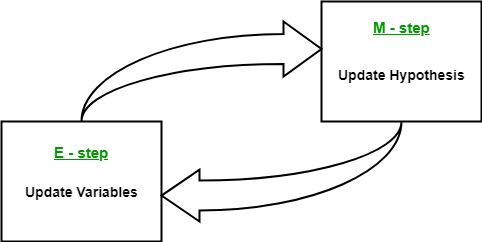Image Source:

👉

## Aim of Expectation-Maximization algorithm

The Expectation-Maximization algorithm aims to use the available observed data of the dataset to estimate the missing data of the latent variables and then using that data to update the values of the parameters in the maximization step.

Let us understand the EM algorithm in a detailed manner:

• Initialization Step: In this step, we initialized the parameter values with a set of initial values, then give the set of incomplete observed data to the system with the assumption that the observed data comes from a specific model i.e, probability distribution.
• Expectation Step: In this step, by using the observed data to estimate or guess the values of the missing or incomplete data. It is used to update the variables.
• Maximization Step: In this step, we use the complete data generated in the “Expectation” step to update the values of the parameters i.e, update the hypothesis.
• Checking of convergence Step: Now, in this step, we checked whether the values are converging or not, if yes, then stop otherwise repeat these two steps i.e, the “Expectation” step and “Maximization” step until the convergence occurs.

## Flow chart for EM algorithm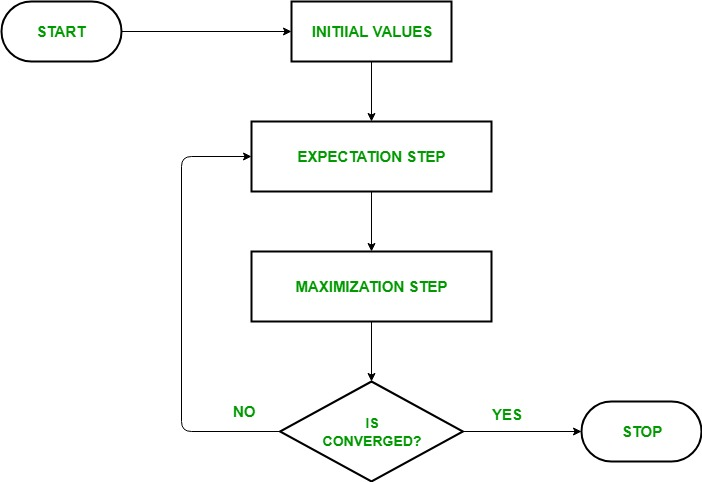Image Source:

• The basic two steps of the EM algorithm i.e, E-step and M-step are often pretty easy for many of the machine learning problems in terms of implementation.
• The solution to the M-steps often exists in the closed-form.
• It is always guaranteed that the value of likelihood will increase after each iteration.

• It has slow convergence.
• It converges to the local optimum only.
• It takes both forward and backward probabilities into account. This thing is in contrast to that of numerical optimization which considers only forward probabilities.

## Applications of EM Algorithm

The latent variable model has several real-life applications in Machine learning:

• 👉 Used to calculate the Gaussian density of a function.
• 👉 Helpful to fill in the missing data during a sample.
• 👉 It finds plenty of use in different domains such as Natural Language Processing (NLP), Computer Vision, etc.
• 👉 Used in image reconstruction in the field of Medicine and Structural Engineering.
• 👉 Used for estimating the parameters of the Hidden Markov Model (HMM) and also for some other mixed models like Gaussian Mixture        Models, etc.
• 👉 Used for finding the values of latent variables.

## Use-Case of EM Algorithm

### Basics of Gaussian Distribution

I’m sure you’re familiar with Gaussian Distributions (or the Normal Distribution) since this distribution is heavily used in the field of Machine Learning and Statistics. It has a bell-shaped curve, with the observations symmetrically distributed around the mean (average) value.

The given image shown has a few Gaussian distributions with different values of the mean (μ) and variance (σ2). Remember that the higher the σ (standard deviation) value more would be the spread along the axis.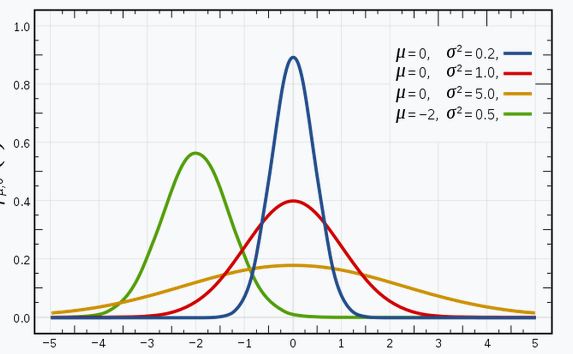Image Source:

In 1-D space, the probability density function of a Gaussian distribution is given by: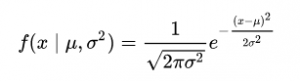Fig. Probability Density Function (PDF)

where μ represents the mean and σ2 represents the variance.

But this would only be true for a variable in 1-D only. In the case of two variables, we will have a 3D bell curve instead of a 2D bell-shaped curve as shown below: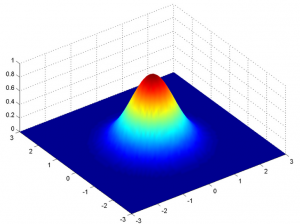The probability density function would be given by: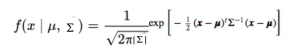where x is the input vector, μ is the 2-D mean vector, and Σ is the 2×2 covariance matrix.  We can generalize the same for the d-dimension.

Thus, for the Multivariate Gaussian model, we have x and μ as vectors of length d, and Σ would be a d x d covariance matrix.

Hence, for a dataset having d features, we would have a mixture of k Gaussian distributions (where k represents the number of clusters), each having a certain mean vector and variance matrix.

But our question is: ” How we can find out the mean and variance for each Gaussian?”

For finding these values, we using a technique called Expectation-Maximization (EM).

### Gaussian Mixture Models

The main assumption of these mixture models is that there are a certain number of Gaussian distributions, and each of these distributions represents a cluster. Hence, a Gaussian Mixture model tries to group the observations belonging to a single distribution together.

Gaussian Mixture Models are probabilistic models which use the soft clustering approach for distributing the observations in different clusters i.e, different Gaussian distribution.

For Example, the Gaussian Mixture Model of 2 Gaussian distributions

We have two Gaussian distributions-  N(𝜇1, 𝜎12) and N(𝜇2, 𝜎22)

Here, we have to estimate a total of 5 parameters:

𝜃 = ( p, 𝜇1, 𝜎12,𝜇2, 𝜎22)

where p is the probability that the data comes from the first  Gaussian distribution and 1-p that it comes from the second Gaussian distribution.

Then, the probability density function (PDF) of the mixture model is given by:

g(x|𝜃) = p g1(x| 𝜇1, 𝜎12) + (1-p)g2(x| 𝜇2, 𝜎22 )

Objective: To best fit a given probability density by finding 𝜃 = ( p, 𝜇1, 𝜎12,𝜇2, 𝜎22) through EM iterations.

## Implementation of GMM in Python

It’s time to dive into the code! Here for implementation, we use the Sklearn Library of Python.

From sklearn, we use the GaussianMixture class which implements the EM algorithm for fitting a mixture of Gaussian models. After object creation, by using the GaussianMixture.fit method we can learns a Gaussian Mixture Model from the training data.

Step-1: Import necessary Packages and create an object of the Gaussian Mixture class

Python Code:

Step-2: Fit the created object on the given dataset

`gmm.fit(np.expand_dims(data, 1))`

Step-3: Print the parameters of 2 input Gaussians

```Gaussian_nr = 1
print('Input Normal_distb {:}: μ = {:.2}, σ = {:.2}'.format("1", Mean1, Standard_dev1))
print('Input Normal_distb {:}: μ = {:.2}, σ = {:.2}'.format("2", Mean2, Standard_dev2))```
```Output:

Input Normal_distb 1: μ = 2.0, σ = 4.0

Input Normal_distb 2: μ = 9.0, σ = 2.0```

Step-4: Print the parameters after mixing of 2 Gaussians

```for mu, sd, p in zip(gmm.means_.flatten(), np.sqrt(gmm.covariances_.flatten()), gmm.weights_):
print('Normal_distb {:}: μ = {:.2}, σ = {:.2}, weight = {:.2}'.format(Gaussian_nr, mu, sd, p))
g_s = stats.norm(mu, sd).pdf(x) * p
plt.plot(x, g_s, label='gaussian sklearn');
Gaussian_nr += 1```

Output:

Normal_distb 1: μ = 1.7, σ = 3.8, weight = 0.61

Normal_distb 2: μ = 8.8, σ = 2.2, weight = 0.39

Step-5: Plot the distribution plots

```sns.distplot(data, bins=20, kde=False, norm_hist=True)
gmm_sum = np.exp([gmm.score_samples(e.reshape(-1, 1)) for e in x])
plt.plot(x, gmm_sum, label='gaussian mixture');
plt.legend();```

Output:

This completes our implementation of GMM!

## End Notes

If you liked this and want to know more, go visit my other articles on Data Science and Machine Learning by clicking on the Link

Something not mentioned or want to share your thoughts? Feel free to comment below And I’ll get back to you.

## Chirag Goyal

Currently, I am pursuing my Bachelor of Technology (B.Tech) in Computer Science and Engineering from the Indian Institute of Technology Jodhpur(IITJ). I am very enthusiastic about Machine learning, Deep Learning, and Artificial Intelligence.

The media shown in this article on Expectation-Maximization Algorithm are not owned by Analytics Vidhya and is used at the Author’s discretion.